# YruRU Method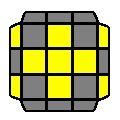##### R U R' U R U' R' U R U2 R'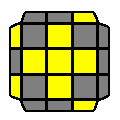##### R U2 R2 U' R2 U' R2 U2 R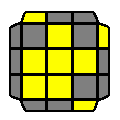##### R U R' U R U2 R'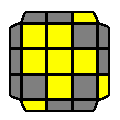##### R' U' R U' R' U2 R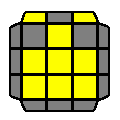##### R' U' R U' R' U2 R2 U R' U R U2 R'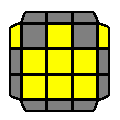##### R U2 R' U' R U' R2 U2 R U R' U R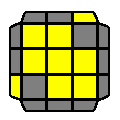##### R U R' U R U' R' U R U' R' U R U2 R'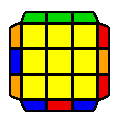##### R U' R U R U R U' R' U' R2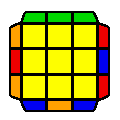##### R2 U R U R' U' R' U' R' U R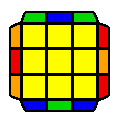##### R2 U2 R U2 R2 U2 R2 U2 R U2 R2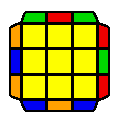##### R' U' R U' R U R U' R' U R U R2 U' R'

Average movecount: 10.7 + 12.0

Average double turns: 2.1 + 3.0

Algorithms: 7 + 4

2GLL is a set which is not common knowledge to everyone, thus while the algorithms are being memorised, this is a 2LLL scheme for cases not yet memorised. This is simply converting all 2GLL cases to O 2GLL (i.e. EPLL) by solving OCLL, and then doing the EPLL algorithm.

Most of 2GLL simply is a combination of 2 sune algorithms and its cyclic shifts, and is much easier to memorise compared to other sets of similar size.